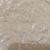Trending ▼   ResFinder# CBSE Class 10 Pre Board 2021 : Mathematics (R. B. K. School (RBK), Mira Road)

6 pages, 52 questions, 0 questions with responses, 0 total responses,30Heena Shah +Fave Message ProfileTimelineUploads
 Home > knowledgetree21 >   F Also featured on: School Page cbse10_pre_boards and 2 moreFormatting page ...

PRELIMINARY EXAMINATION II (2020-21) SUBJECT: MATHEMATICS Grade: X Date :11/01/2021 Time: 3 hours Total Marks: 80 General Instructions: 1. This question paper contains two parts A and B. 2. Both Part A and Part B have internal choices. Part A: 1. It consists of three sections- I and II. 2. Section I has 16 questions of 1 mark each. Internal choice is provided in 5 questions. 3. Section II has 4 questions on case study. Each case study has 5 case-based sub-parts. An examinee is to attempt any 4 out of 5 sub-parts. Part B: 1. Question No 21 to 26 are Very short answer Type questions of 2 mark each, 2. Question No 27 to 33 are Short Answer Type questions of 3 marks each 3. Question No 34 to 36 are Long Answer Type questions of 5 marks each. 4. Internal choice is provided in 2 questions of 2 marks, 2 questions of 3 marks and 1 question of 5 marks. Q.No PART A Marks Section-I Section I has 16 questions of 1 mark each. Internal choice is provided in 5 questions. 1) Show that 13/3125 has terminating decimal expansion 1 2) If the sum of zeros of the quadratic polynomial 3x2+kx-5 is 4, then find the value of k. 1 3) For what value of k, the pair of linear equations 2x + 3y = 7 and 5x + ky = 9 has a unique solution? 1 4) If ab = 1050 and LCM of a and b is 70, then find the HCF (a,b) 1 5) Is 301 a term of 5,11,17,23,......? 1 6) Find the value of p for which the equation px2 - 8x + 4 = 0 has two equal roots 1 7) Write a quadratic polynomial whose sum of zeros is 6 and product is 9. 1 8) The angle between the tangent and the radius drawn at the point of contact is ______ 1 9) Area of a sector is of the area of the circle. Find the degree measure of its minor arc. 1 10) If the mid-point of the line segment joining the points P(6 , b-2) and Q(-2 , 4 ) is (2 , -3 ), find the value of b. 1Formatting page ...Formatting page ...Formatting page ...Formatting page ...Formatting page ...